# Intro to the Viterbi Algorithm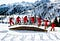Photo by Jeffrey Brandjes on Unsplash

In the last post on Hidden Markov models (HMM), we never solved the problem of finding the most probable sequence of coins used. If you didn’t read the post on HMMs, I would highly encourage you to do so. For those of you who did not, I’ll outline the problem. Let’s say some guru came up to you and told you to pick a coin from a bag (there are only two coins in the bag) and flip the coin. You’ll either observe a head or a tail. You then put the coin back in the bag and perform the…

# Intro to Hidden Markov Models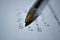Photo by Antoine Dautry on Unsplash

In the last post, we went over Expectation-Maximization (EM). In the example I gave for EM, we have some prior distributions of 2 coins. The coins were placed in a bag and randomly selected from the bag. While we didn’t know what coin was selected, we wanted still to attempt to find the most likely estimate of the probability of heads. Rather than attempting to find the most likely estimate of the probability of heads, what if we wanted to find the most likely sequence of coins for some sequence of flips. …

# Intro to Expectation Maximization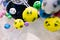Photo by dylan nolte on Unsplash

I’ve written a few posts on parameter estimation. The first post was on Maximum-Likelihood Estimation (MLE) where we want to find the value of some parameter θ renders the training data most likely, this could also be known as the parameter which is most likely given the training data. Bayesian Parameter Estimation (BPE) expands on MLE as prior information about θ is either given or assumed. What if the data is not complete? What if there is missing data, where missing could imply noise, lost, or hidden data? This is where Expectation-Maximization (EM) comes in. The EM algorithm is an…

# Intro to Principal Component Analysis (PCA) in Python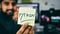https://unsplash.com/photos/D9Zow2REm8U

Imagine you are tackling a classification problem where you are classifying apples and oranges. You have a dataset with a large number of features, but you know that only a few features matter. Subjectively, you could manually reduce the dataset to the features you think would yield good results while still maintaining a large amount of information that differentiates an apple from an orange. Alternatively, we could use an unbiased solution, math, to reduce the dimensionality of the dataset. In this post, we will be going over Principal Component Analysis (PCA). PCA is an algorithm used for dimensionality reduction.

PCA…

# Intro to Bayesian Parameter Estimation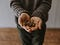https://unsplash.com/photos/TBHOuN6URGU

# What is Bayesian Parameter Estimation?

Remember Maximum Likelihood Estimate (MLE) from the last post? In MLE, we assume that the training data is a good representation of the population data. What if we have prior information? How can we utilize this prior information in parameter estimation? This is where Bayesian Parameter Estimation comes in. In Bayesian Parameter Estimation, θ is a random variable where prior information about θ is either given or assumed. We update the prior assumption/knowledge based on the new training samples. …

# Intro to Maximum Likelihood Estimate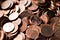https://unsplash.com/photos/6tG_liBojOk

Note: This post will be a bit more math-heavy than my other posts.

Imagine flipping a penny 10 times. After those 10 times, you get heads 4 times. Your friend flips the coin 10 times and gets heads all 10 times. You and your friend keep flipping the coin, but out of all the trials, you get a probability of heads being 65%. Your friend argues that this is wrong and the true probability is 50%, but what if the coin is biased? Given these trials, how would we go about finding the most likely equation (or estimate) for the…

# Bayes’ Theorem made Simple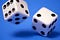https://unsplash.com/photos/uKlneQRwaxY

# Bayes’ Theorem

Conditional probability is inescapable in machine learning, but it doesn’t often come in the form of the conditional probability formula from the last blog post. Usually, if we want to find P(A|B), we will know how to calculate P(B|A). So…how can we get P(A|B) from P(B|A)? This is where Bayes’ theorem comes in.

# Conditional Probability made Simple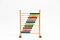https://unsplash.com/photos/cHhbULJbPwM?utm_source=unsplash&utm_medium=referral&utm_content=creditShareLink

Conditional probability was one of the most complex topics for me to grasp as an undergrad, so I thought I would try to make it easier. I figured there would be no better way to learn conditional probability than to teach it by using something I think we all love…pizza. So, why should you care about conditional probability? Well, conditional probability is used everywhere in machine learning. Say we want to classify whether an image is a cat of a dog. We are just solving if P( Dog | Image ) > P( Cat | Image ). There are many…

# Probabilistic Decision Making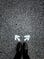https://unsplash.com/photos/PXB7yEM5LVs

This is the first post in a series of posts in which we will be going over using probability and statistics to better influence decision making. In this post, we will go over the benefits of using probability to rationalize decisions.

# Probabilistic Decision Making in its Simplest Form

Given a bag where we have 6 apples and 4 orange with replacement (placing fruit back in the bag), we can say that the probability of an apple is 60% and the probability of an orange is 40%. So, with just this information, if we were to create some machine learning classifier, the optimal decision would be to always…## Paul Butler

Master's student, programmer, and data science enthusiast